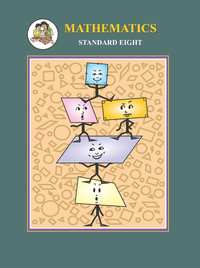Share

# Balbharati solutions for Maharashtra state board Class 8 Mathematics chapter 16 - Surface area and Volume [Latest edition]

Textbook page

#### Chapters## Chapter 16: Surface area and Volume

Practice Set 16.1Practice Set 16.2Practice Set 16.3

#### Balbharati solutions for Maharashtra state board Class 8 Mathematics Chapter 16 Surface area and Volume Exercise Practice Set 16.1 [Pages 108 - 109]

Practice Set 16.1 | Q 1 | Page 108

Find the volume of a box if its length, breadth and height are 20 cm, 10.5 cm and 8 cm respectively.

Practice Set 16.1 | Q 2 | Page 108

A cuboid shape soap bar has volume 150 cc. Find its thickness if its length is 10 cm and breadth is 5 cm.

Practice Set 16.1 | Q 3 | Page 108

How many bricks of length 25 cm, breadth 15 cm and height 10 cm are required to build a wall of length 6 m, height 2.5 m and breadth 0.5 m?

Practice Set 16.1 | Q 4 | Page 109

For rain water harvesting a tank of length 10 m, breadth 6 m and depth 3 m is built. What is the capacity of the tank? How many litre of water can it hold?

#### Balbharati solutions for Maharashtra state board Class 8 Mathematics Chapter 16 Surface area and Volume Exercise Practice Set 16.2 [Pages 110 - 111]

Practice Set 16.2 | Q 1 | Page 110

In each example given below, radius of base of a cylinder and its height are given. Then find the curved surface area and total surface area.
(1) r = 7 cm, h = 10 cm
(2) r = 1.4 cm, h = 2.1 cm
(3) r = 2.5 cm, h = 7 cm
(4) r = 70 cm, h = 1.4 cm
(5) r = 4.2 cm, h = 14 cm

Practice Set 16.2 | Q 2 | Page 110

Find the total surface area of a closed cylindrical drum if its diameter is 50 cm and height is 45 cm. (π = 3.14)

Practice Set 16.2 | Q 3 | Page 111

Find the area of base and radius of a cylinder if its curved surface area is 660 sqcm and height is 21 cm.

Practice Set 16.2 | Q 4 | Page 111

Find the area of the sheet required to make a cylindrical container which is open at one side and whose diameter is 28 cm and height is 20 cm. Find the approximate area of the sheet required to make a lid of height 2 cm for this container.

#### Balbharati solutions for Maharashtra state board Class 8 Mathematics Chapter 16 Surface area and Volume Exercise Practice Set 16.3 [Page 112]

Practice Set 16.3 | Q 1 | Page 112

Find the volume of the cylinder if height (h) and radius of the base (r) are as given below.
(1) r = 10.5 cm, h =8 cm
(2) r = 2.5 m, h = 7 m
(3) r = 4.2 cm, h = 5 cm
(4) r = 5.6 cm, h = 5 cm

Practice Set 16.3 | Q 2 | Page 112

How much iron is needed to make a rod of length 90 cm and diameter 1.4 cm?

Practice Set 16.3 | Q 3 | Page 112

How much water will a tank hold if the interior diameter of the tank is 1.6 m and its depth is 0.7 m?

Practice Set 16.3 | Q 4 | Page 112

Find the volume of the cylinder if the circumference of the cylinder is 132 cm and height is 25 cm.

## Chapter 16: Surface area and Volume

Practice Set 16.1Practice Set 16.2Practice Set 16.3## Balbharati solutions for Maharashtra state board Class 8 Mathematics chapter 16 - Surface area and Volume

Balbharati solutions for Maharashtra state board Class 8 Mathematics chapter 16 (Surface area and Volume) include all questions with solution and detail explanation. This will clear students doubts about any question and improve application skills while preparing for board exams. The detailed, step-by-step solutions will help you understand the concepts better and clear your confusions, if any. Shaalaa.com has the Maharashtra State Board Maharashtra state board Class 8 Mathematics solutions in a manner that help students grasp basic concepts better and faster.

Further, we at Shaalaa.com provide such solutions so that students can prepare for written exams. Balbharati textbook solutions can be a core help for self-study and acts as a perfect self-help guidance for students.

Concepts covered in Maharashtra state board Class 8 Mathematics chapter 16 Surface area and Volume are Standard Unit of Volume, Surface Area of Cylinder, Volume of a Cylinder, Euler’S Formula.

Using Balbharati Class 8 solutions Surface area and Volume exercise by students are an easy way to prepare for the exams, as they involve solutions arranged chapter-wise also page wise. The questions involved in Balbharati Solutions are important questions that can be asked in the final exam. Maximum students of Maharashtra State Board Class 8 prefer Balbharati Textbook Solutions to score more in exam.

Get the free view of chapter 16 Surface area and Volume Class 8 extra questions for Maharashtra state board Class 8 Mathematics and can use Shaalaa.com to keep it handy for your exam preparation